Newton's Law of Gravitation

Idea: Newton's Universal Law of Gravitation states that any two objects exert a gravitational force of attraction on each other. The direction of the force is along the line joing the objects (See Fig.(7.3)). The magnitude of the force is proportional to the product of the gravitational masses of the objects, and inversely proportional to the square of the distance between them. For the two objects in Figure 7.3: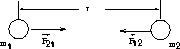Figure 7.3: Gravitational Force Between Two Masses

•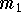exerts a force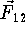on.
•exerts a forceon.
• By Newton's third law:
•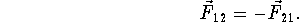• The magnitude of the gravitational force is:
•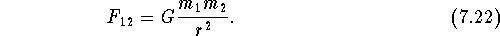• G is Newton's constant:
•Note:

• The inertial mass of an object determines the amount of force needed to produce a given acceleration of that object. The gravitational mass determines the force of gravitational attraction between two bodies. In Newtonian mechanics, these two masses have no obvious connection with each other. Nonetheless, it was observed empirically that they are numerically equal. This remarkable fact was known for centuries, but remained unexplained until Einstein's General Theory of relativity.
• Newton's gravitational constant is extremely small when expressed in terms of laboratory sized objects: the gravitational force between twoobjects separated by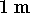is only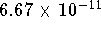Newtons.
• For an object of mass m near the Earth's surface:
•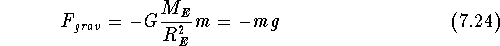where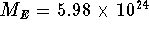kg is the mass of the Earth and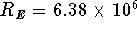m is the radius of the earth and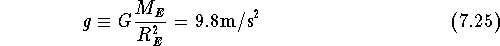in agreement with the expression in Chapter 3.

Definition: Gravitional Potential Energy

Due to the gravitational force of attraction, any two objects with massesandlocated a distance r apart have the ability to do work. Hence they have potential energy. The gravitational potential energy of such objects is: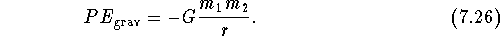Note:

• Recall that only differences in potential energy are physically relevant. In the above, the zero of gravitational potential energy has been arbitrarily chosen to be zero at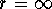. i.e. when the objects are infinitely far apart.
• The negative sign is a consequence of the attractive nature of the gravitational force. When the objects are far apart, the gravitational force naturally moves them closer, decreasing their potential energy (i.e. making it more negative).
• Gravitational potential energy near Earth's surface: For an object of mass m a distance h above the earth's surface:
•Ifwe can approximate: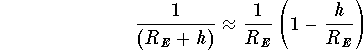so that:This agrees with the expression used in Chapter 5.